# How to Calculate and Solve for Cold Crushing Strength | Refractories

The cold crushing strength is illustrated by the image below.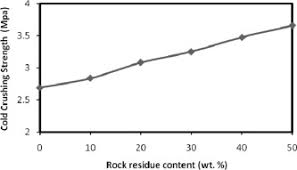To compute for cold crushing strength, two essential parameters are needed and these parameters are Maximum Load (MI) and Cross-sectional Area (A).

The formula for calculating cold crushing strength:

CCS = MI/A

Where:

CCS = Cold Crushing Strength
A = Cross-sectional Area

Let’s solve an example;
Find the cold crushing strength when the maximum load is 44 and the cross-sectional area is 11.

This implies that;

MI = Maximum Load = 44
A = Cross-sectional Area = 11

CCS = MI/A
CCS = 44/11
CCS = 4

Therefore, the cold crushing strength is 4 Pa.

Calculating the Maximum Load when the Cold Crushing Strength and the Cross-Sectional Area is Given.

MI = CCS x A

Where:

CCS = Cold Crushing Strength
A = Cross-sectional Area

Let’s solve an example;
Given that the cold crushing strength is 15 and the cross-sectional area is 5. Find the maximum load?

This implies that;

CCS = Cold Crushing Strength = 15
A = Cross-sectional Area = 5

MI = CCS x A
MI = 15 x 5
MI = 75

Therefore, the cold crushing strength is 75.

Calculating the Cross-Sectional Area when the Cold Crushing Strength and the Maximum Load is Given.

A = MI / CCS

Where:

A = Cross-sectional Area
CCS = Cold Crushing Strength

Let’s solve an example;
Find the cross-sectional area when the cold crushing strength is 10 and the maximum load is 30.

This implies that;

CCS = Cold Crushing Strength = 10
MI = Maximum Load = 30

A = MI / CCS
A = 30 / 10
A = 3

Therefore, the cross-sectional area is 3.

Nickzom Calculator – The Calculator Encyclopedia is capable of calculating the cold crushing strength.

To get the answer and workings of the cold crushing strength using the Nickzom Calculator – The Calculator Encyclopedia. First, you need to obtain the app.

You can get this app via any of these means:

You can also try the demo version via https://www.nickzom.org/calculator

Apple (Paid) – https://itunes.apple.com/us/app/nickzom-calculator/id1331162702?mt=8
Once, you have obtained the calculator encyclopedia app, proceed to the Calculator Map, then click on Materials and Metallurgical under Engineering.Now, Click on Refractories under Materials and MetallurgicalNow, Click on Cold Crushing Strength under Refractories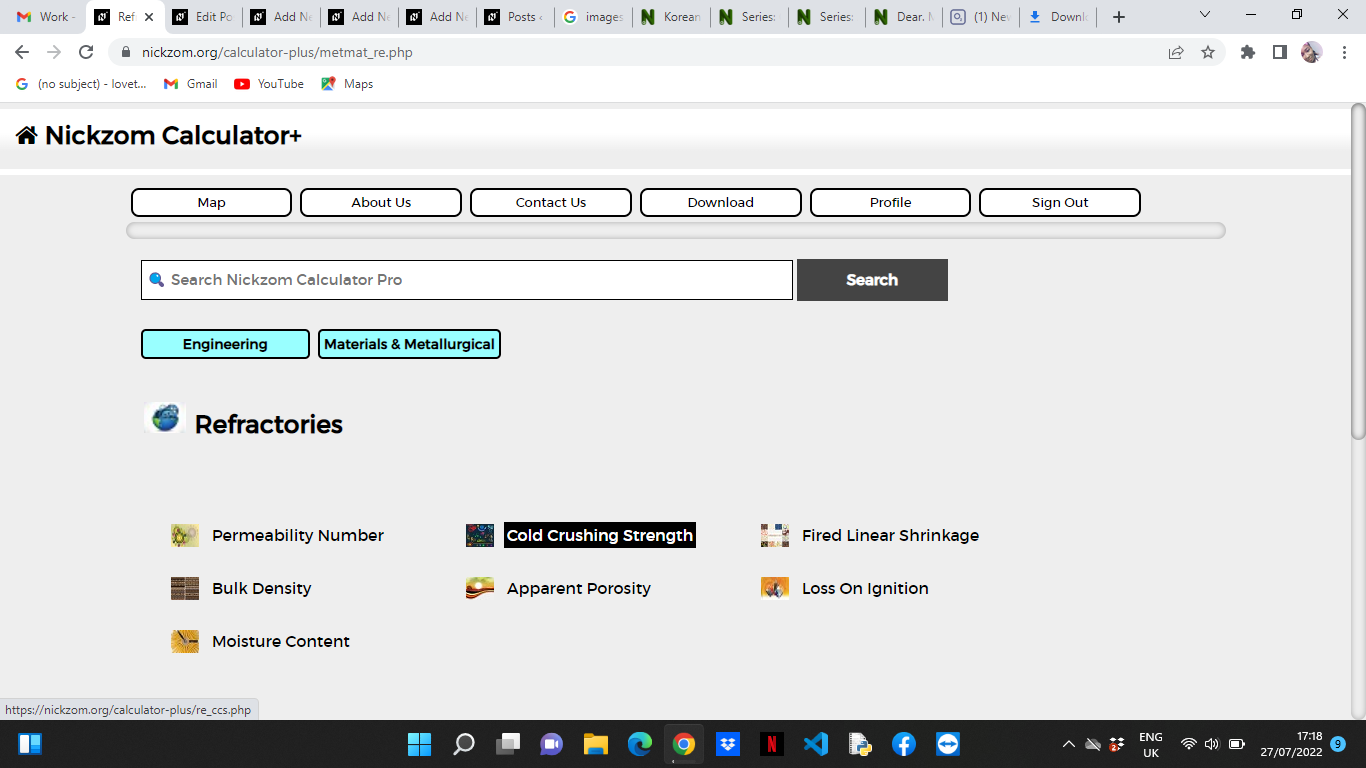The screenshot below displays the page or activity to enter your values, to get the answer for the cold crushing strength according to the respective parameter which is the Maximum Load (MI) and Cross-sectional Area (A).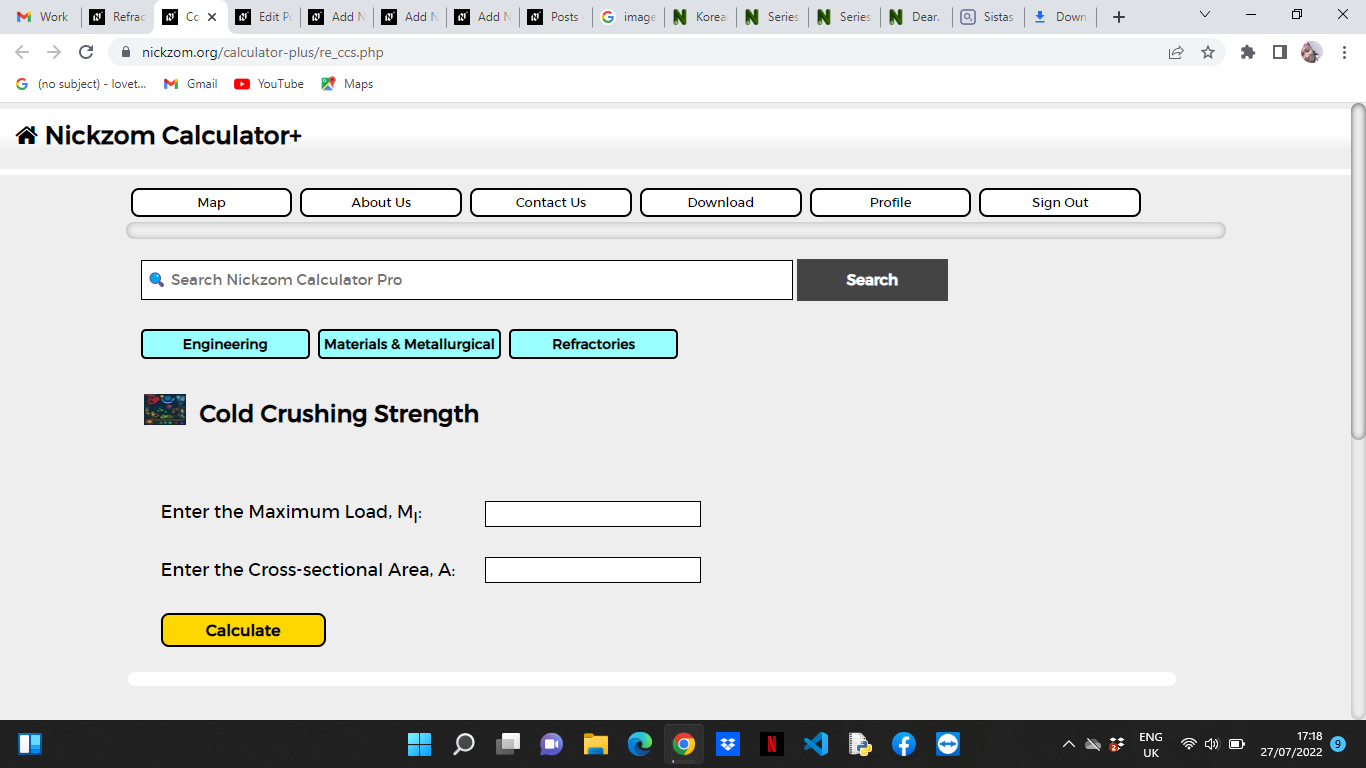Now, enter the values appropriately and accordingly for the parameters as required by the Maximum Load (MI) is 44 and Cross-sectional Area (A) is 11.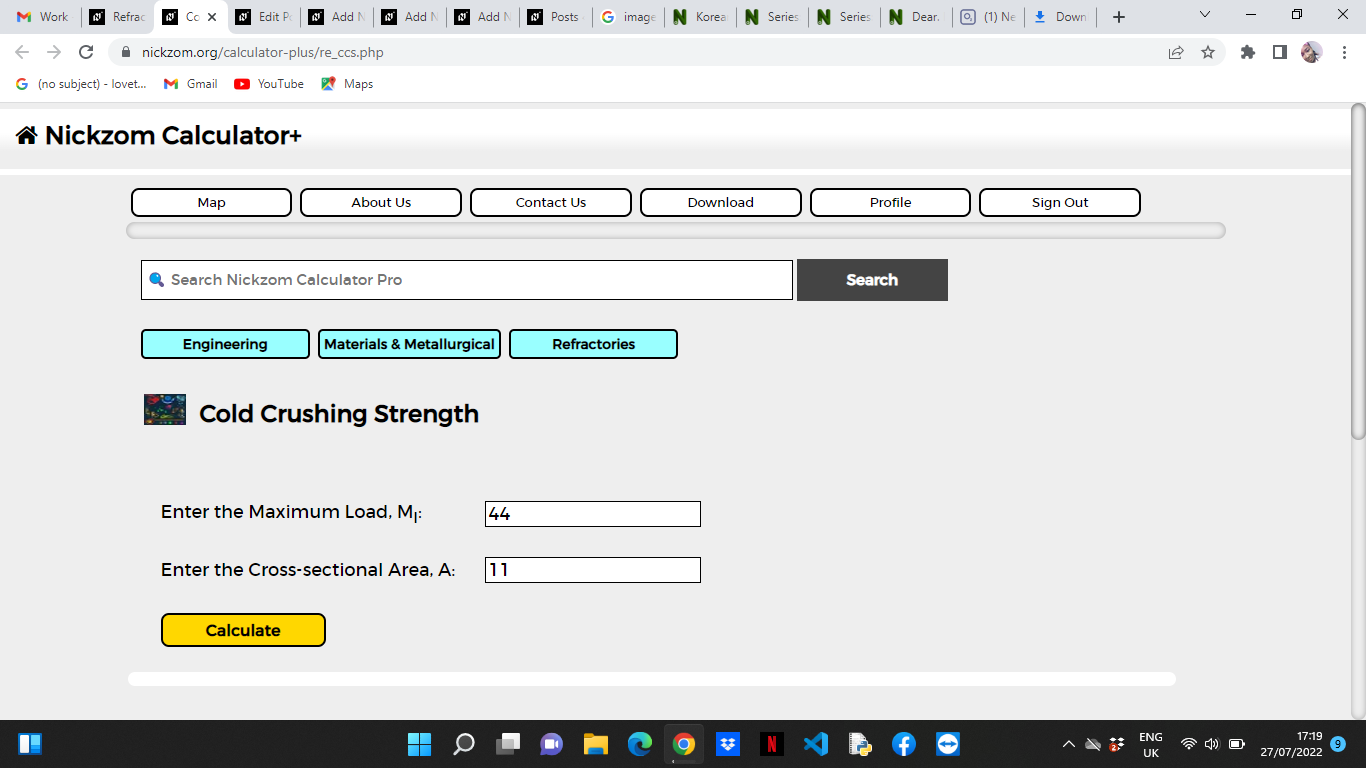Finally, Click on Calculate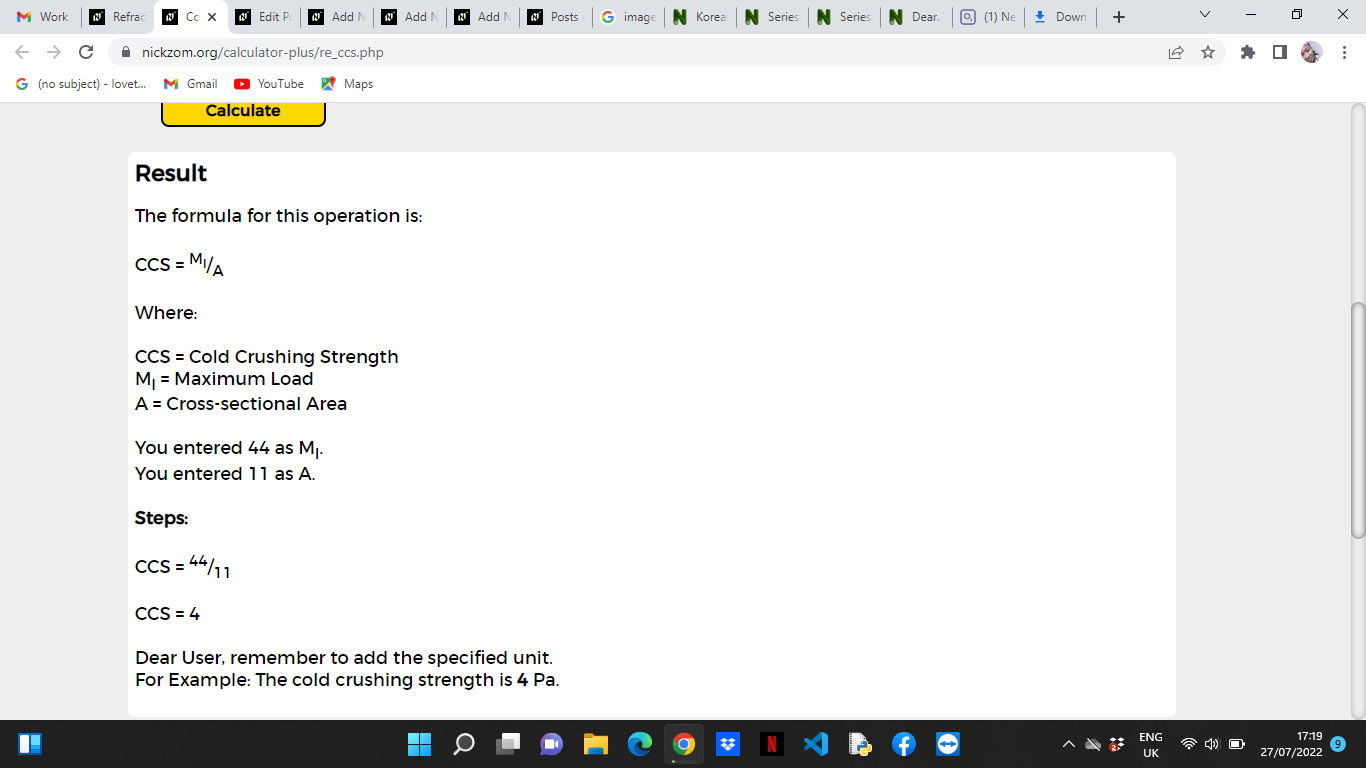As you can see from the screenshot above, Nickzom Calculator– The Calculator Encyclopedia solves for the cold crushing strength and presents the formula, workings and steps too.